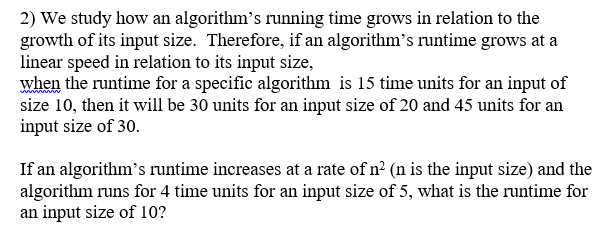# Answered! We study how an algorithm's running time grows in relation to the growth of its input size. Therefore, if an…We study how an algorithm’s running time grows in relation to the growth of its input size. Therefore, if an algorithm’s runtime grows at a linear speed in relation to its input size, when the runtime for a specific algorithm is 15 time units for an input of size 10, then it will be 30 units for an input size of 20 and 45 units for an input size of 30. If an algorithm’s runtime increases at a rate of n (n is the input size and the algorithm runs for 4 time units for an input size of 5, what is the runt for an input size of 10?